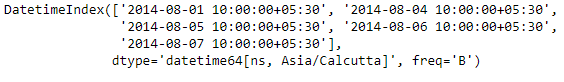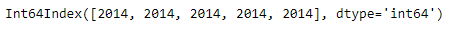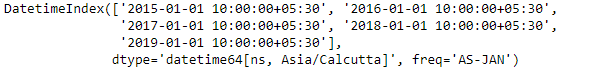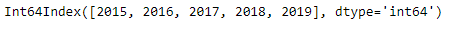# Python | Pandas DatetimeIndex.year

Python is a great language for doing data analysis, primarily because of the fantastic ecosystem of data-centric python packages. Pandas is one of those packages and makes importing and analyzing data much easier.

Pandas` DatetimeIndex.year` attribute outputs an Index object containing the value of years present in the Datetime object.

Syntax: DatetimeIndex.year

Return: Index containing years.

Example #1: Use `DatetimeIndex.year` attribute to find the years present in the DatetimeIndex.

 `# importing pandas as pd ` `import` `pandas as pd ` ` `  `# Create the DatetimeIndex ` `# Here the 'B' represents Business day frequency ` `didx ``=` `pd.DatetimeIndex(start ``=``'2014-08-01 10:00'``, freq ``=``'B'``,  ` `                            ``periods ``=` `5``, tz ``=``'Asia/Calcutta'``) ` ` `  `# Print the DatetimeIndex ` `print``(didx) `

Output :Now we want to find all the year values present in the DatetimeIndex object.

 `# find all the years in the object ` `didx.year `

Output :As we can see in the output, the function has returned an Index object containing the year value of each entry in the DatetimeIndex object.
&n bsp;
Example #2: Use `DatetimeIndex.year` attribute to find the years present in the DatetimeIndex.

 `# importing pandas as pd ` `import` `pandas as pd ` ` `  `# Create the DatetimeIndex ` `# Here the 'AS' represents Year start frequency ` `didx ``=` `pd.DatetimeIndex(start ``=``'2014-08-01 10:00'``, freq ``=``'AS'``,  ` `                             ``periods ``=` `5``, tz ``=``'Asia/Calcutta'``) ` ` `  `# Print the DatetimeIndex ` `print``(didx) `

Output :Now we want to find all the year values present in the DatetimeIndex object.

 `# find all the years in the object ` `didx.year `

Output :As we can see in the output, the function has returned an Index object containing the year value of each entry in the DatetimeIndex object.

Attention geek! Strengthen your foundations with the Python Programming Foundation Course and learn the basics.

To begin with, your interview preparations Enhance your Data Structures concepts with the Python DS Course.

My Personal Notes arrow_drop_upCheck out this Author's contributed articles.

If you like GeeksforGeeks and would like to contribute, you can also write an article using contribute.geeksforgeeks.org or mail your article to contribute@geeksforgeeks.org. See your article appearing on the GeeksforGeeks main page and help other Geeks.

Please Improve this article if you find anything incorrect by clicking on the "Improve Article" button below.

Article Tags :

Be the First to upvote.

Please write to us at contribute@geeksforgeeks.org to report any issue with the above content.